# 拆解式解读如何用飞桨复现胶囊神经网络（Capsule Network）

2020/08/30 13:42Dynamic Routing Between Capsules是 NIPS 2017的一篇论文。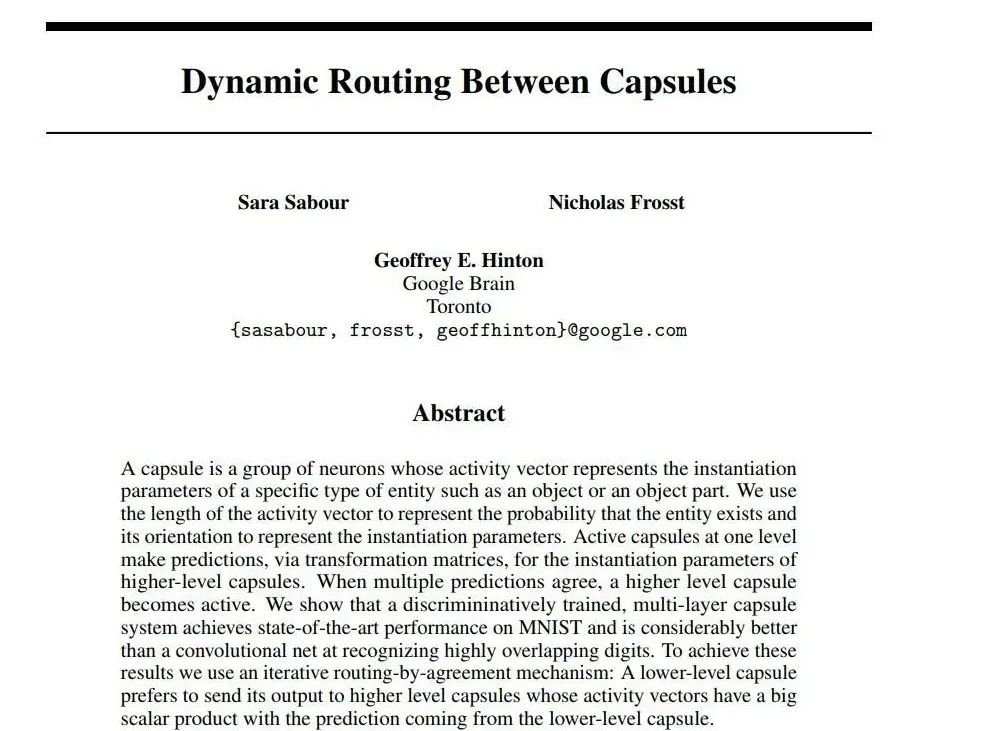下载安装命令

## CPU版本安装命令

## GPU版本安装命令
pip install -f https://paddlepaddle.org.cn/pip/oschina/gpu paddlepaddle-gpu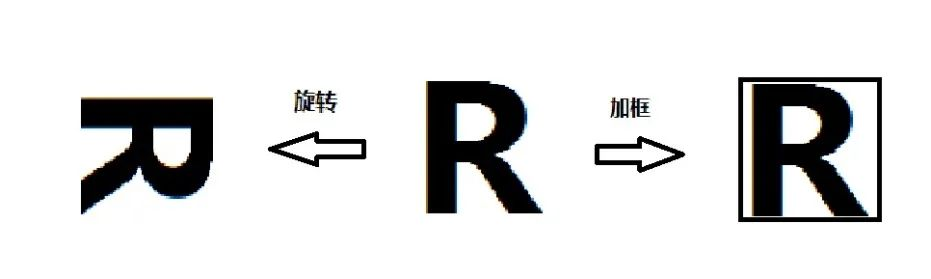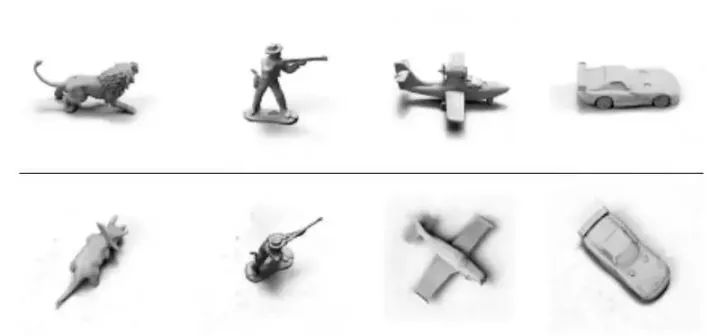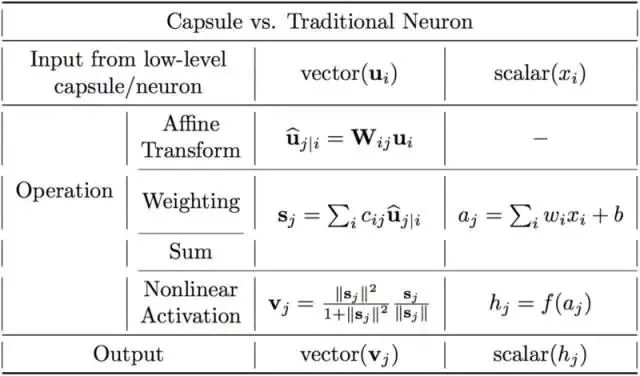1. 输入标量的标量加权： wixi+b

2. 加权输入标量之和：aj = ∑iwixi+b

3. 标量到标量的非线性变换：hj = f(aj)

1. 输入向量的矩阵乘法：ûj|I = Wijui

2. 输入向量的标量加权： cijûj|I

3. 加权输入向量之和： sj = ∑i cijûj|I

4. 向量到向量的非线性变换：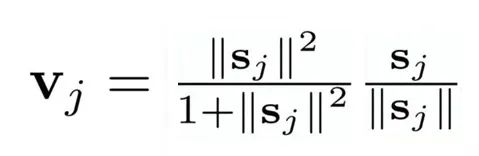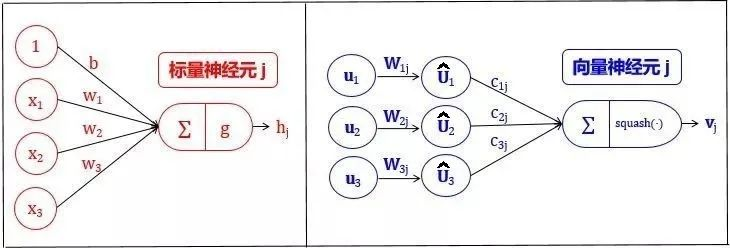1. 输入向量的矩阵乘法

2. 输入向量的标量加权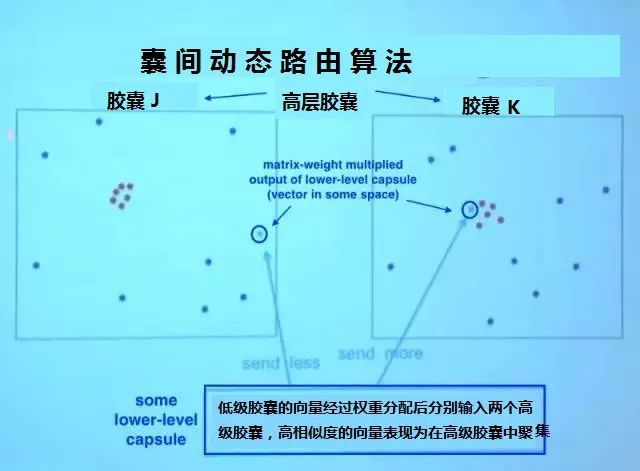1. 权重均为非负标量。

2. 对每个低层胶囊i而言，所有权重的总和等于1（经过softmax函数加权）。

3. 对每个低层胶囊i而言，权重的数量等于高层胶囊的数量。

4. 这些权重的数值由迭代动态路由算法确定。

3. 加权输入向量之和

4. 向量到向量的非线性变换

CapsNet的另一大创新是新颖的非线性激活函数，这个函数接受一个向量，然后在不改变方向的前提下，压缩它的长度到1以下。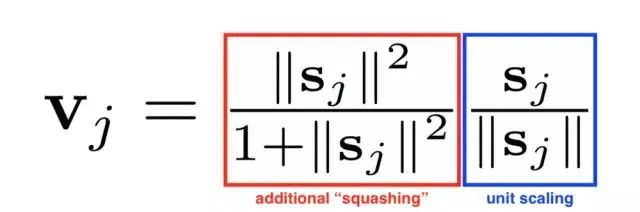def squash(self,vector):
'''
压缩向量的函数，类似激活函数，向量归一化
Args:
vector：一个4维张量 [batch_size,vector_num,vector_units_num,1]
Returns:
一个和x形状相同，长度经过压缩的向量
输入向量|v|（向量长度）越大，输出|v|越接近1
'''
vec_abs = fluid.layers.sqrt(fluid.layers.reduce_sum(fluid.layers.square(vector)))
scalar_factor = fluid.layers.square(vec_abs) / (1 + fluid.layers.square(vec_abs))
vec_squashed = scalar_factor * fluid.layers.elementwise_div(vector, vec_abs)
return(vec_squashed)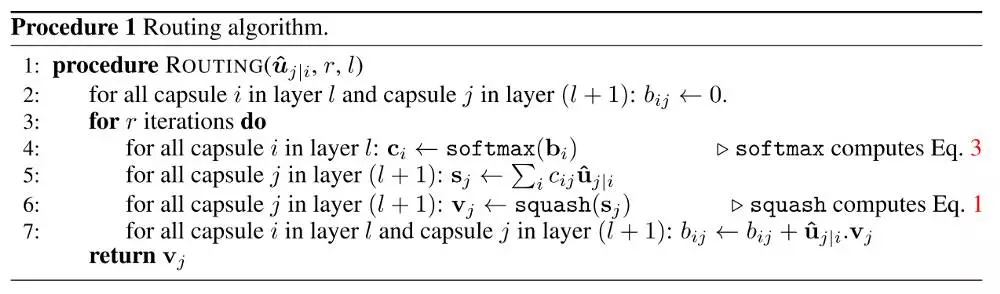• 伪代码的第一行指明了算法的输入：低层输入向量经过矩阵乘法得到的û，以及路由迭代次数r。最后一行指明了算法的输出，高层胶囊的向量Vj。

• 第2行的bij是一个临时变量，存放了低层向量对高层胶囊的权重，它的值会在迭代过程中逐个更新，当开始一轮迭代时，它的值经过softmax转换成cij。在囊间动态路由算法开始时，bij的值被初始化为零(但是经过softmax后会转换成非零且各个权重相等的cij)。

• 第3行表明第4-7行的步骤会被重复r次（路由迭代次数）。

• 第4行计算低层胶囊向量的对应所有高层胶囊的权重。bi的值经过softmax后会转换成非零权重ci且其元素总和等于1。

• 如果是第一次迭代，所有系数cij的值会相等。例如，如果我们有8个低层胶囊和10个高层胶囊，那么所有cij的权重都将等于0.1。这样初始化使不确定性达到最大值：低层胶囊不知道它们的输出最适合哪个高层胶囊。当然，随着这一进程的重复，这些均匀分布将发生改变。

• 第5行，那里将涉及高层胶囊。这一步计算经前一步确定的路由系数加权后的输入向量的总和，得到输出向量sj。

• 第7行进行更新权重，这是路由算法的精髓所在。我们将每个高层胶囊的向量vj与低层原来的输入向量û逐元素相乘求和获得内积（也叫点积，点积检测胶囊的输入和输出之间的相似性（下图为示意图）），再用点积结果更新原来的权重bi。这就达到了低层胶囊将其输出发送给具有类似输出的高层胶囊的效果，刻画了向量之间的相似性。这一步骤之后，算法跳转到第3步重新开始这一流程，并重复r次。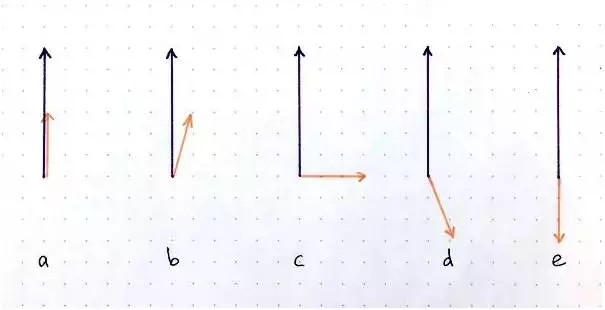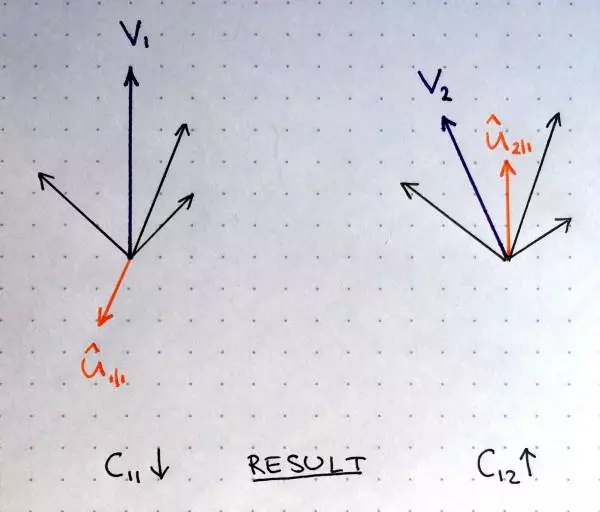▲ 点积运算即为向量的内积（点积）运算，

class Capsule_Layer(fluid.dygraph.Layer):
def __init__(self,pre_cap_num,pre_vector_units_num,cap_num,vector_units_num):
'''
胶囊层的实现类，可以直接同普通层一样使用
Args:
pre_vector_units_num(int):输入向量维度
vector_units_num(int):输出向量维度
pre_cap_num(int)：输入胶囊数
cap_num(int)：输出胶囊数
routing_iters(int):路由迭代次数，建议3次
Notes:
胶囊数和向量维度影响着性能,可作为主调参数
'''
super(Capsule_Layer,self).__init__()
self.routing_iters = 3
self.pre_cap_num = pre_cap_num
self.cap_num = cap_num
self.pre_vector_units_num = pre_vector_units_num
for j in range(self.cap_num):
input_dim=pre_vector_units_num,output_dim=vector_units_num))

def squash(self,vector):
'''
压缩向量的函数，类似激活函数，向量归一化
Args:
vector：一个4维张量 [batch_size,vector_num,vector_units_num,1]
Returns:
一个和x形状相同，长度经过压缩的向量
输入向量|v|（向量长度）越大，输出|v|越接近1
'''
vec_abs = fluid.layers.sqrt(fluid.layers.reduce_sum(fluid.layers.square(vector)))
scalar_factor = fluid.layers.square(vec_abs) / (1 + fluid.layers.square(vec_abs))
vec_squashed = scalar_factor * fluid.layers.elementwise_div(vector, vec_abs)
return(vec_squashed)

def capsule(self,x,B_ij,j,pre_cap_num):
'''
这是动态路由算法的精髓。
Args:
x：输入向量,一个四维张量 shape = (batch_size,pre_cap_num,pre_vector_units_num,1)
B_ij: shape = (1,pre_cap_num,cap_num,1)路由分配权重，这里将会选取(split)其中的第j组权重进行计算
j：表示当前计算第j个胶囊的路由
pre_cap_num:输入胶囊数
Returns:
v_j:经过多次路由迭代之后输出的4维张量（单个胶囊）
B_ij：计算完路由之后又拼接(concat)回去的权重
Notes:
B_ij,b_ij,C_ij,c_ij注意区分大小写哦
'''
x = fluid.layers.reshape(x,(x.shape,pre_cap_num,-1))
u_hat = getattr(self,'u_hat_w'+str(j))(x)
u_hat = fluid.layers.reshape(u_hat,(x.shape,pre_cap_num,-1,1))
shape_list = B_ij.shape#(1,1152,10,1)
split_size = [j,1,shape_list-j-1]
for i in range(self.routing_iters):
C_ij = fluid.layers.softmax(B_ij,axis=2)
b_il,b_ij,b_ir = fluid.layers.split(B_ij,split_size,dim=2)
c_il,c_ij,b_ir = fluid.layers.split(C_ij,split_size,dim=2)
v_j = fluid.layers.elementwise_mul(u_hat,c_ij)
v_j = fluid.layers.reduce_sum(v_j,dim=1,keep_dim=True)
v_j = self.squash(v_j)
v_j_expand = fluid.layers.expand(v_j,(1,pre_cap_num,1,1))
u_v_produce = fluid.layers.elementwise_mul(u_hat,v_j_expand)
u_v_produce = fluid.layers.reduce_sum(u_v_produce,dim=2,keep_dim=True)
b_ij += fluid.layers.reduce_sum(u_v_produce,dim=0,keep_dim=True)
B_ij = fluid.layers.concat([b_il,b_ij,b_ir],axis=2)
return v_j,B_ij

def forward(self,x):
'''
Args:
x:shape = (batch_size,pre_caps_num,vector_units_num,1) or (batch_size,C,H,W)
如果是输入是shape=(batch_size,C,H,W)的张量，
则将其向量化shape=(batch_size,pre_caps_num,vector_units_num,1)
满足:C * H * W = vector_units_num * caps_num
其中 C >= caps_num
Returns:
capsules:一个包含了caps_num个胶囊的list
'''
if x.shape!=1:
x = fluid.layers.reshape(x,(x.shape,self.pre_cap_num,-1))
temp_x = fluid.layers.split(x,self.pre_vector_units_num,dim=2)
temp_x = fluid.layers.concat(temp_x,axis=1)
x = fluid.layers.reshape(temp_x,(x.shape,self.pre_cap_num,-1,1))
x = self.squash(x)
B_ij = fluid.layers.ones((1,x.shape,self.cap_num,1),dtype='float32')/self.cap_num#
capsules = []
for j in range(self.cap_num):
cap_j,B_ij = self.capsule(x,B_ij,j,self.pre_cap_num)
capsules.append(cap_j)
capsules = fluid.layers.concat(capsules,axis=1)
return capsules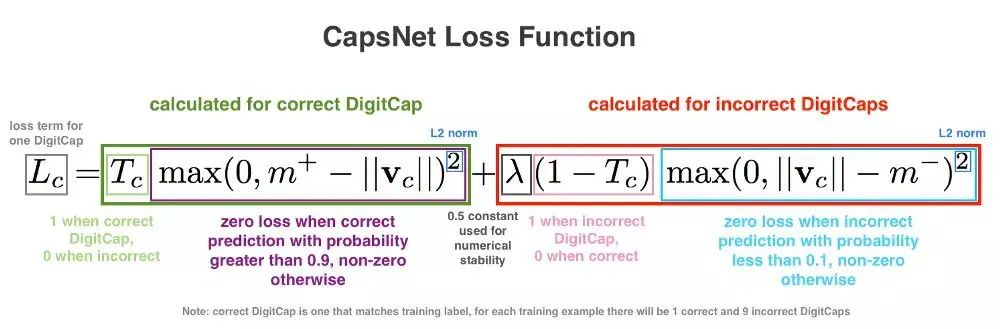|v|为胶囊输出向量的模长，一定程度上表示了类概率的大小，我们再拟定一个量m，用这个变量来衡量概率是否合适。公式右项包括了一个lambda系数以确保训练中的数值稳定性（lambda为固定值0.5），这两项取平方是为了让损失函数符合L2正则。

 def get_loss_v(self,label):
'''
计算边缘损失
Args:
label:shape=(32,10) one-hot形式的标签
Notes:
这里我调用Relu把小于0的值筛除
m_plus：正确输出项的概率（|v|）大于这个值则loss为0，越接近则loss越小
m_det：错误输出项的概率（|v|）小于这个值则loss为0，越接近则loss越小
（|v|即胶囊(向量)的模长）
'''
#计算左项，虽然m+是单个值，但是可以通过广播的形式与label（32,10)作差
max_l =  fluid.layers.relu(train_params['m_plus'] - self.output_caps_v_lenth)
#平方运算后reshape
max_l = fluid.layers.reshape(fluid.layers.square(max_l),(train_params['batch_size'],-1))#32,10
#同样方法计算右项
max_r =  fluid.layers.relu(self.output_caps_v_lenth - train_params['m_det'])
max_r = fluid.layers.reshape(fluid.layers.square(max_r),(train_params['batch_size'],-1))#32,10
#合并的时候直接用one-hot形式的标签逐元素乘算便可
margin_loss = fluid.layers.elementwise_mul(label,max_l)\
+ fluid.layers.elementwise_mul(1-label,max_r)*train_params['lambda_val']
self.margin_loss = fluid.layers.reduce_mean(margin_loss,dim=1)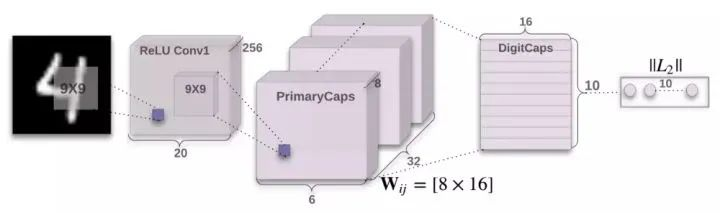1. 输入图片28x28首先经过1x256x9x9的卷积层 获得256个20x20的特征图；

2. 用8组256x32x9x9(stride=2)的卷积获得8组32x6x6的特征图；

3. 将获取的特征图向量化输入10个胶囊，这10个胶囊输出向量的长度就是各个类别的概率。

class Capconv_Net(fluid.dygraph.Layer):
def __init__(self):
super(Capconv_Net,self).__init__()
for i in range(8):

def forward(self,x,v_units_num):
x = getattr(self,'conv0')(x)
capsules = []
for i in range(v_units_num):
temp_x = getattr(self,'conv_vector_'+str(i))(x)
capsules.append(fluid.layers.reshape(temp_x,(train_params['batch_size'],-1,1,1)))
x = fluid.layers.concat(capsules,axis=2)        x = self.squash(x)
return x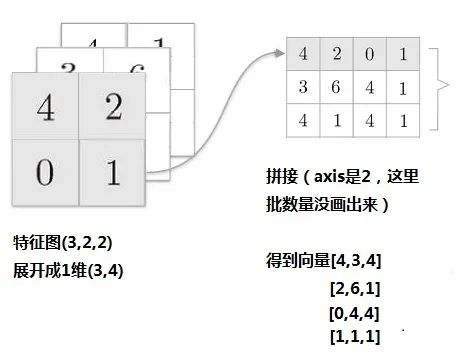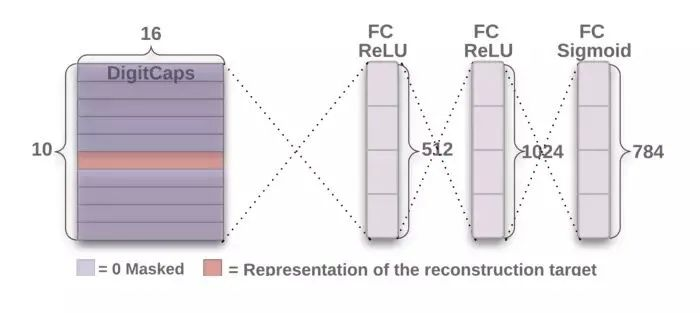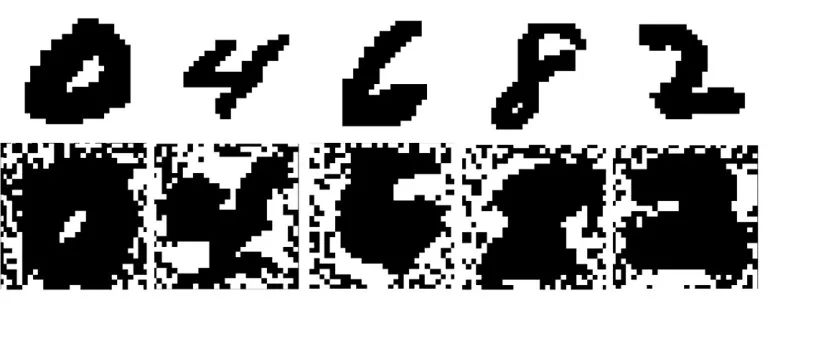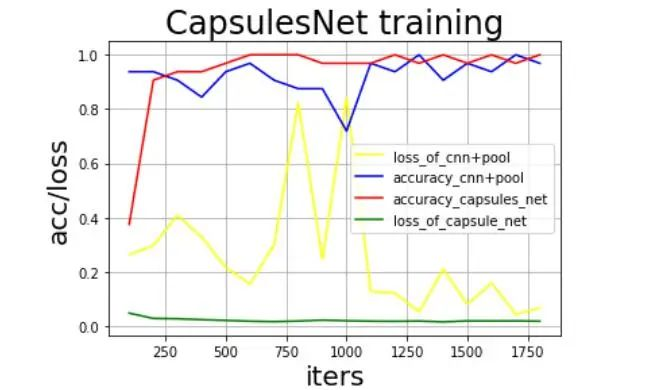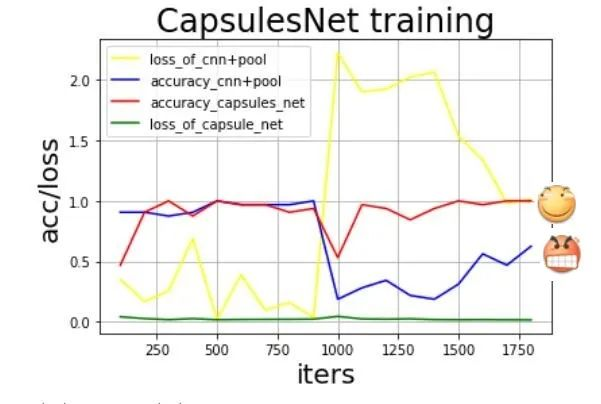Capsule显露了它处理不同位姿的本领！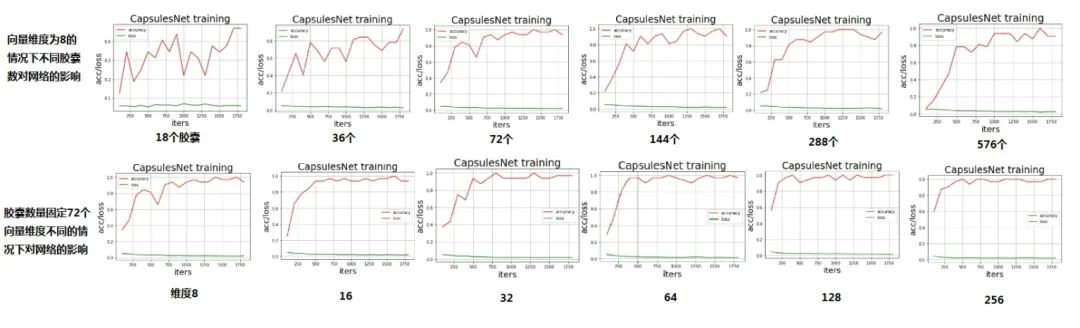https://aistudio.baidu.com/aistudio/projectdetail/657114?shared=1

下载安装命令

## CPU版本安装命令

## GPU版本安装命令### 作者的其它热门文章

0
1 收藏

0 评论
1 收藏
0